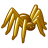# How to automate creating points a certain distance and angle from central point

7921
2
01-13-2013 09:10 AMNew Contributor
Hi! I have conducted bird surveys from a particular location  (centroid of grid cell). I have data of the direction (N, NE, NW etc.) from the location and also the distance. I want to be able to automate the mapping of the point locations of birds I heard. My table has the location of the centroid for the observation which is constant (lat and long) and also has a distance (25ft, 50ft etc) and direction field (0 - 360 degrees) for each bird I identified by sound. I am treating these observations as points. I want each point to be mapped around the centroid - understanding that I will have overlap.

Do you know of any way to automate this since I have 700 observations?

Also, am I on the right track with the distance/direction tool IF I were to do it by hand?

Thanks so much,
Lori
Tags (1)
2 RepliesRegular Contributor III

If you comfortable with python you could do it with python Writing geometries—Help | ArcGIS for Desktop

If your not comfortable with code see this thread Moving a point by distance and bearingbyMVP Legendary Contributor

Let us do it python school... because you can do it directly as a spreadsheet or you can use Numpyarrayto featureclass or the insertcursor approach to produce your geometries after the calculations are done.  Verbose description.

Do it in reverse first

``````import numpy as np
np.set_printoptions(edgeitems=5,linewidth=80,precision=4,suppress=True,threshold=10)
cent = np.array([0,0],dtype="float64")
pnts = np.array([[1,1],[1,2],[-3,3],[-4,-4],[5,-5]],dtype="float64")
Xs = pnts[:,0]
Ys = pnts[:,1]
diff = pnts-cent
angles= np.degrees(np.arctan2(diff[:,1],diff[:,0]))
dist = np.hypot(diff[:,0],diff[:,1])
data = np.vstack((Xs,Ys,angles,dist)).T
print("Origin (0,0)\n   {:<10s} {:<10s} {:<10s} {:<10s}".format("Xs","Ys","angles","dist"))
print("{}".format(data))
‍‍‍‍‍‍‍‍‍‍‍‍``````

Origin (0,0)

Xs         Ys         angles     dist

[[   1.        1.       45.        1.4142]

[   1.        2.       63.4349    2.2361]

[  -3.        3.      135.        4.2426]

[  -4.       -4.     -135.        5.6569]

[   5.       -5.      -45.        7.0711]]

Now lets do it forward

``````# Now only distance and angle are known.  Calculate the point coordinates from distance and angle
angles= np.degrees(np.arctan2(diff[:,1],diff[:,0]))
dist = np.hypot(diff[:,0],diff[:,1])
Xn = sines * dist
Yn = coses * dist
data = np.vstack((angles,dist,Xs,Ys)).T
print("Origin (0,0)\n  {:<10s} {:<10s} {:<10s} {:<10s}".format("angles","dist","Xn","Yn"))
print("{}".format(data))
‍‍‍‍‍‍‍‍‍‍‍``````

Origin (0,0)

angles     dist       Xn         Yn

[[  45.        1.4142    1.        1.    ]

[  63.4349    2.2361    1.        2.    ]

[ 135.        4.2426   -3.        3.    ]

[-135.        5.6569   -4.       -4.    ]

[ -45.        7.0711    5.       -5.    ]]

if you can assemble the origin point and all the destination points together into one file, you can then steal the code from the following line to generate your polylines or simply use the toolbox to generate desire lines connect the destinations to the origins

http://www.arcgis.com/home/item.html?id=6ce9db93533345e49350d30a07fc913a

Or there is Bearing Distance To Line—Help | ArcGIS for Desktop which can be use on or better still

XY To Line—Help | ArcGIS for Desktop

generate this....

final from Xc,Yc,Xs,Ys

[[ 0.  0.  1.  1.]

[ 0.  0.  1.  2.]

[ 0.  0. -3.  3.]

[ 0.  0. -4. -4.]

[ 0.  0.  5. -5.]]

from this...

``````N = len(Xs)
Xc = np.empty(N)
Xc.fill(0.) # coincidently 0,0 is used as the center
Yc = np.empty(N)
Yc.fill(0.)
pairs = np.array(list(zip(Xc,Yc,Xs,Ys)))
print("final from Xc,Yc,Xs,Ys\n{}".format(pairs))
‍‍‍‍‍‍‍``````